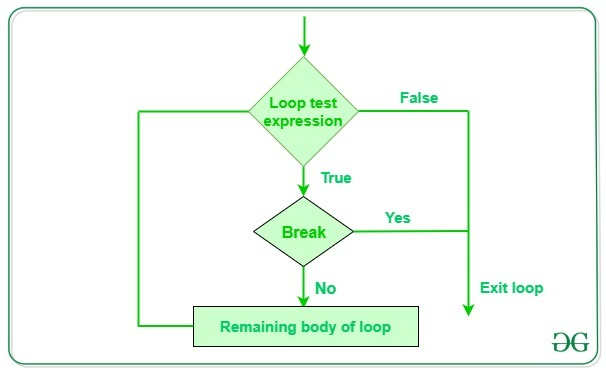# Python break statement

• Difficulty Level : Medium
• Last Updated : 13 Jun, 2022

Using loops in Python automates and repeats the tasks in an efficient manner. But sometimes, there may arise a condition where you want to exit the loop completely, skip an iteration or ignore some statements of the loop before continuing further in the loop. These can be done by loop control statements called jump statements. Loop control or jump statements change execution from its normal sequence. When execution leaves a scope, all automatic objects that were created in that scope are destroyed. Python supports the following control/jump statements.

• continue statement
• break statement
• pass statement

In this article, the main focus will be on the break statement.

Break statement

break statement in Python is used to bring the control out of the loop when some external condition is triggered. break statement is put inside the loop body (generally after if condition).  It terminates the current loop, i.e., the loop in which it appears, and resumes execution at the next statement immediately after the end of that loop. If the break statement is inside a nested loop, the break will terminate the innermost loop.Syntax:

`break`

Example#1:

## Python3

 `# Python program to` `# demonstrate break statement`   `s ``=` `'geeksforgeeks'` `# Using for loop` `for` `letter ``in` `s:`   `    ``print``(letter)` `    ``# break the loop as soon it sees 'e'` `    ``# or 's'` `    ``if` `letter ``=``=` `'e'` `or` `letter ``=``=` `'s'``:` `        ``break`   `print``(& quot` `       ``Out of ``for` `loop & quot` `       ``)` `print``()`   `i ``=` `0`   `# Using while loop` `while` `True``:` `    ``print``(s[i])`   `    ``# break the loop as soon it sees 'e'` `    ``# or 's'` `    ``if` `s[i] ``=``=` `'e'` `or` `s[i] ``=``=` `'s'``:` `        ``break` `    ``i ``+``=` `1`   `print``(& quot` `       ``Out of ``while` `loop & quot` `       ``)`

Output:

```g
e
Out of for loop

g
e
Out of while loop```

In the above example, both the loops are iterating the string ‘geeksforgeeks’ and as soon as they encounter the character ‘e’ or ‘s’, the if the condition becomes true and the flow of execution is brought out of the loop.

Example#2:

## Python3

 `num ``=` `0` `for` `i ``in` `range``(``10``):` `    ``num ``+``=` `1` `    ``if` `num ``=``=` `8``:` `        ``break` `    ``print``(``"The num has value:"``, num)` `print``(``"Out of loop"``)`

Output

```The num has value: 1
The num has value: 2
The num has value: 3
The num has value: 4
The num has value: 5
The num has value: 6
The num has value: 7
Out of loop
```

In the above example, after iterating till num=7, the value of num will be 8 and the break is encountered so the flow of the execution is brought out of the loop.

My Personal Notes arrow_drop_up
Recommended Articles
Page :